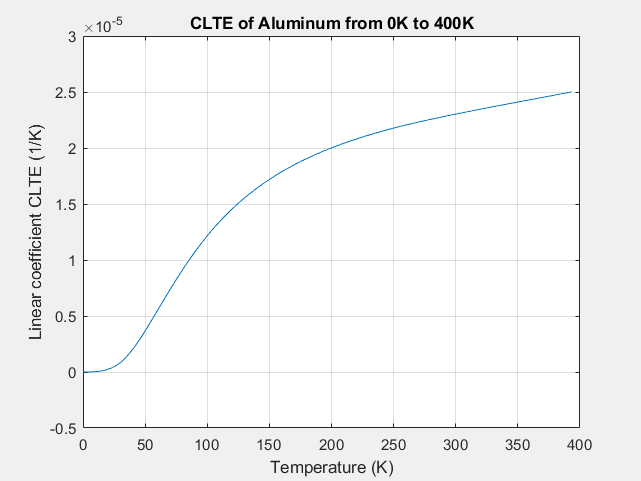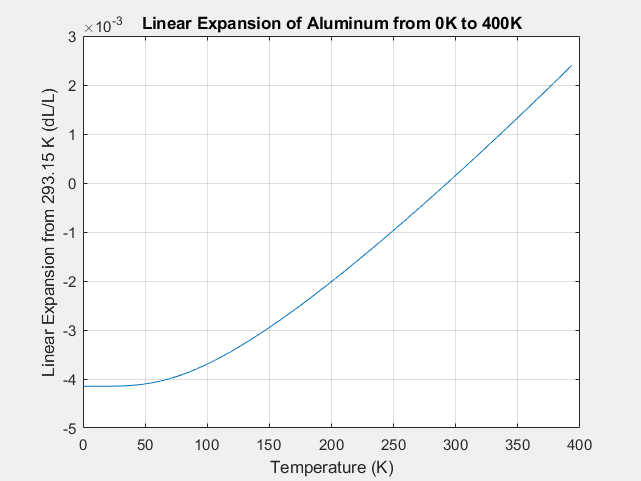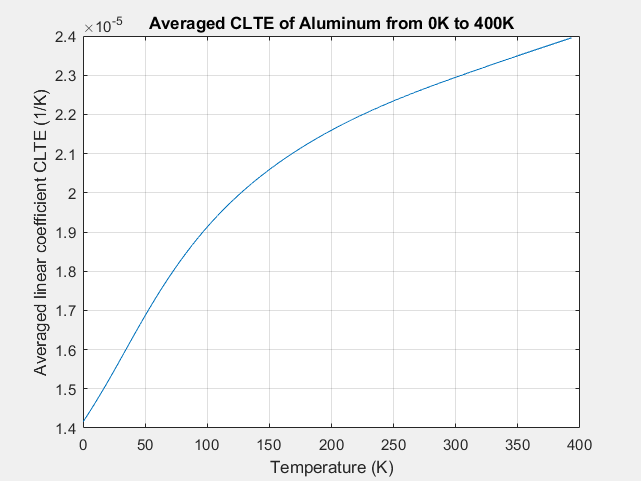# Temperature dependent Thermal expansion coefficient via table

Hi to all,

I try to simulate the thermal expansion as a function of the body’s temperature.

The device under test is an aluminum rod with a length of 1m and a diameter of 0.05m.

The test environment is Thermomechanical (transient, Dynamic) with boundary conditions (fixed support on one end of the rod and fixed temperature values for all faces) or (elastic support on one end of the rod with remote displacement (0) and fixed temperature values for all faces).

Reference data for the CTE of aluminum are taken from:

Absolute linear thermal‐expansion measurements on copper and aluminum from 5 to 320 K

F. R. Kroeger and C. A. Swenson, Journal of Applied Physics 48, 853 (1977)

The CTE for aluminum was set to 22.87e-6 K^-1 at a reference temperature of 293.15 K

The initial temperature was also 293.15 K, the end temperature was set to 140 K

So one can calculate a delta L to -3.5025 mm, which is in very good agreement with the simulation of -3.5055 mm (<1% error).

When using a table with a temperature dependent CTE according the citation given above, the results shows significant differences to the analytical model.

In the citation the CTE(T) was measured with dilatometry and the CTE were was described as a piecewise power series with coefficients taken from least squares approximation of the measured data.

When I import a CTE(T) table and repeat the calculation the results shows a significant difference to the calculated value (3.11 mm shrinking with the analytically equation and about 2.5 mm with the simulation).

Best Regards to all

Hi @bhils and sorry for the delayed response!

My colleague Richard shared this link with me: [SOLVED]Temperature dependent thermal expansion / Code_Aster usage / Code_Aster forums (so instead of putting the “instantaneous” values you should use the average ones from reference temp to the definition temp).

Try that and let us know if it worked out!

Jousef

Hi jousefm,
Where can I change these properties?

Hi @bhils!

You have to simply upload other data, on the page I have posted you can see example data sets.

Best,

Jousef

Hi jousefm,

thanks for your effort → the problem is fixed.

I took the CTE data from the citation, calculated the length change due to the temperature and from these data the averaged CTE. The simulation matches the expected values. I put some graphs in these post. The reference temperature Tref=293.15K.CTE of AluminumCalculated expansion (the integral of the CTE)The averaged CTE used.

Maybe this is helpfull for somebody else.

You can find the CTE Table in the project cited above.

Best regards to all

2 Likes

Marked as the solution, perfectly explained!Would be happy to see your results as well!!

Best,

Jousef